US\$

#### Cart

Home>Men's Shoes>Men's Flats & Loafers>Men Loafers Boat Flats Formal Shoes

# Men Loafers Boat Flats Formal Shoes

US\$ 44.99
Color
Shoe Size
10 11 12 12.5 13 6 6.5 7 8 8.5 9.5
• Detail

US5.5 = EU37 = 235mm = 9.25in (blote voeten lengte)
US6.0 = EU38 = 240mm = 9.45in
US6.5 = EU39 = 245mm = 9.65in
US7.0 = EU40 = 250mm = 9.84in
US8.0 = EU41 = 255mm = 10.04in
US8.5 = EU42 = 260mm = 10.24in
US9.5 = EU43 = 265mm = 10.43in
US10.0 = EU44 = 270mm = 10.63in
US11.0 = EU45 = 275mm = 10.83in
US12.0 = EU46 = 280mm = 11.02in
US12.5 = EU47 = 285mm = 11.22in
US13.0 = EU48 = 290mm = 11.42in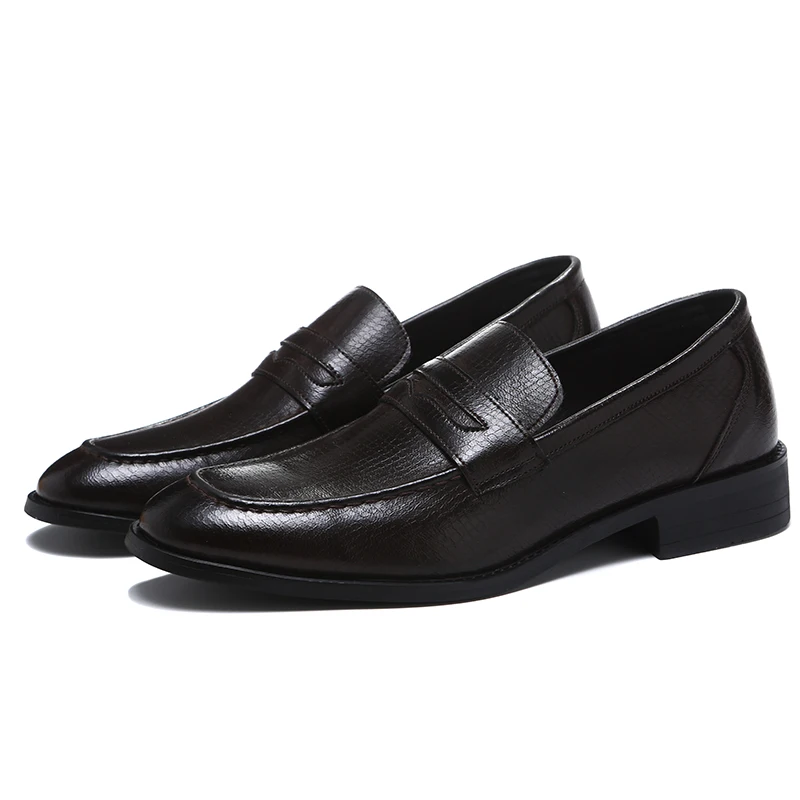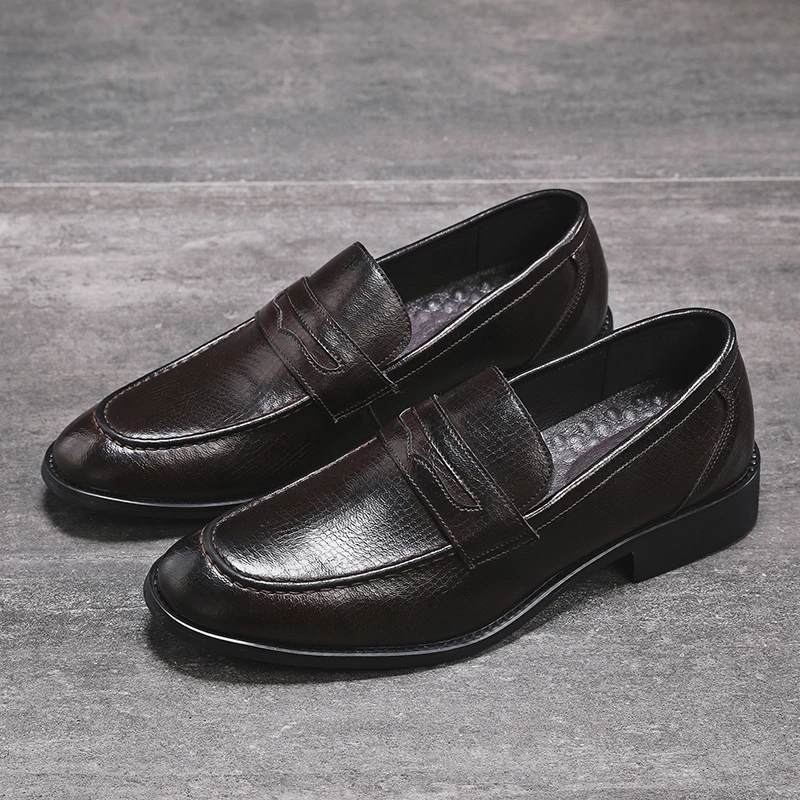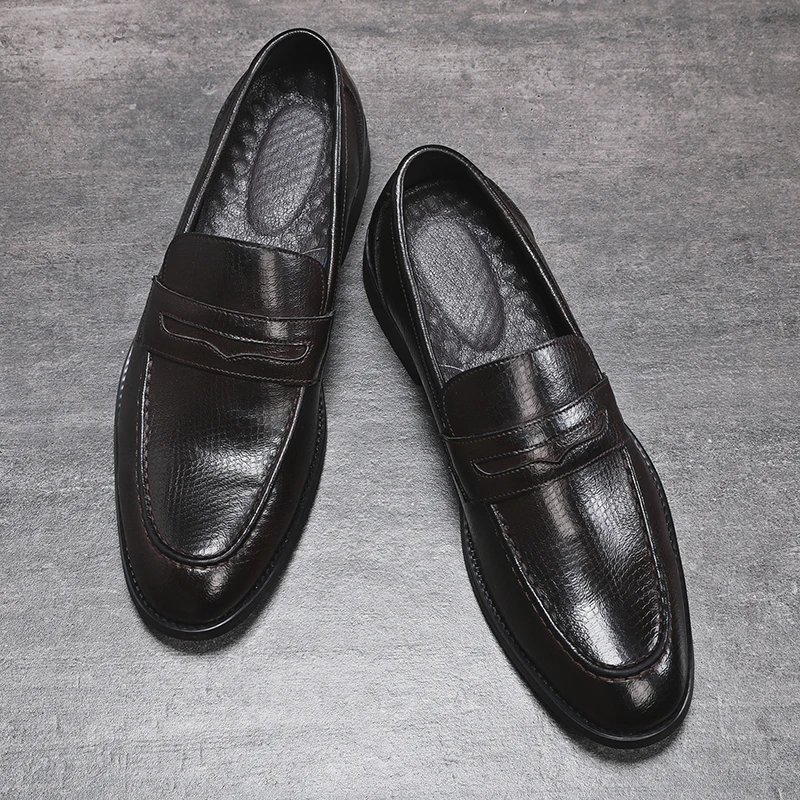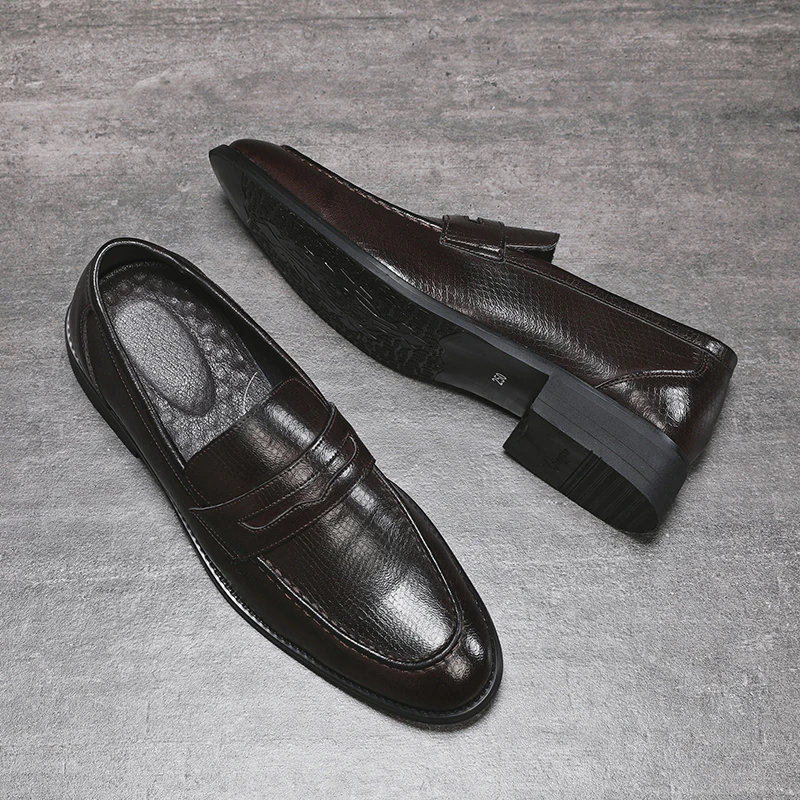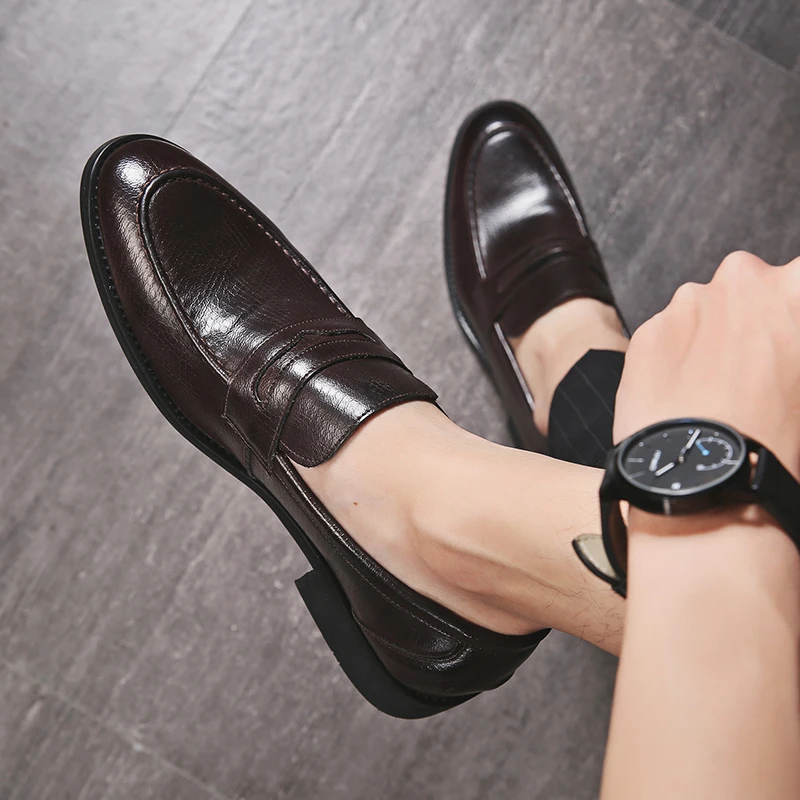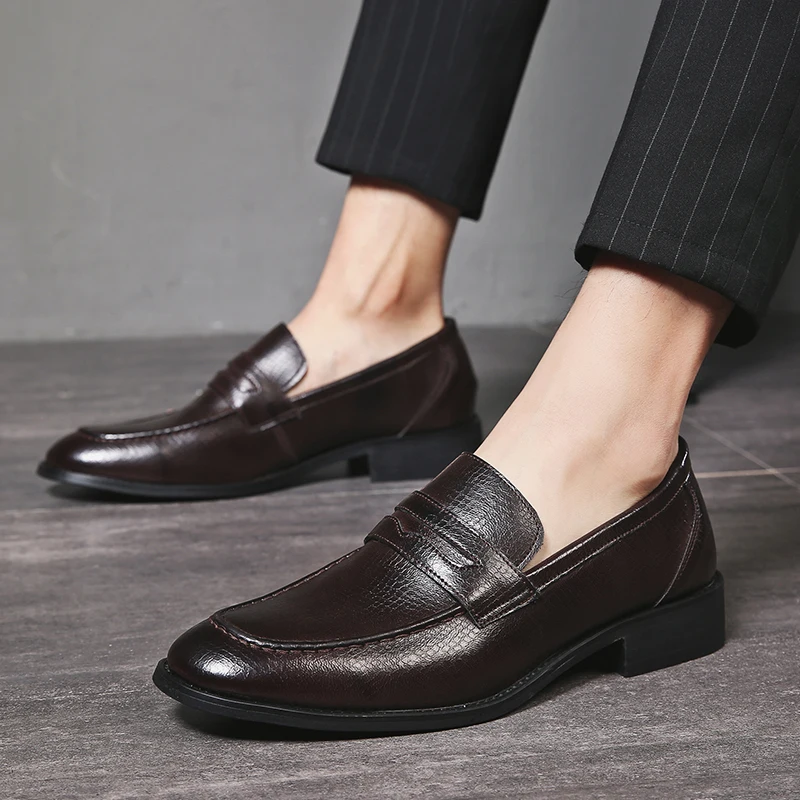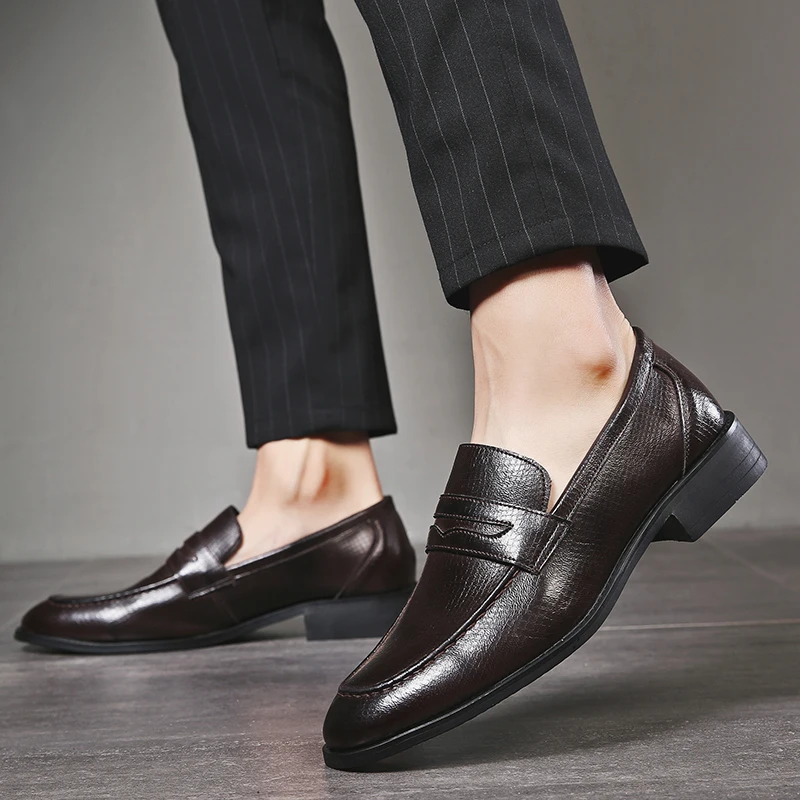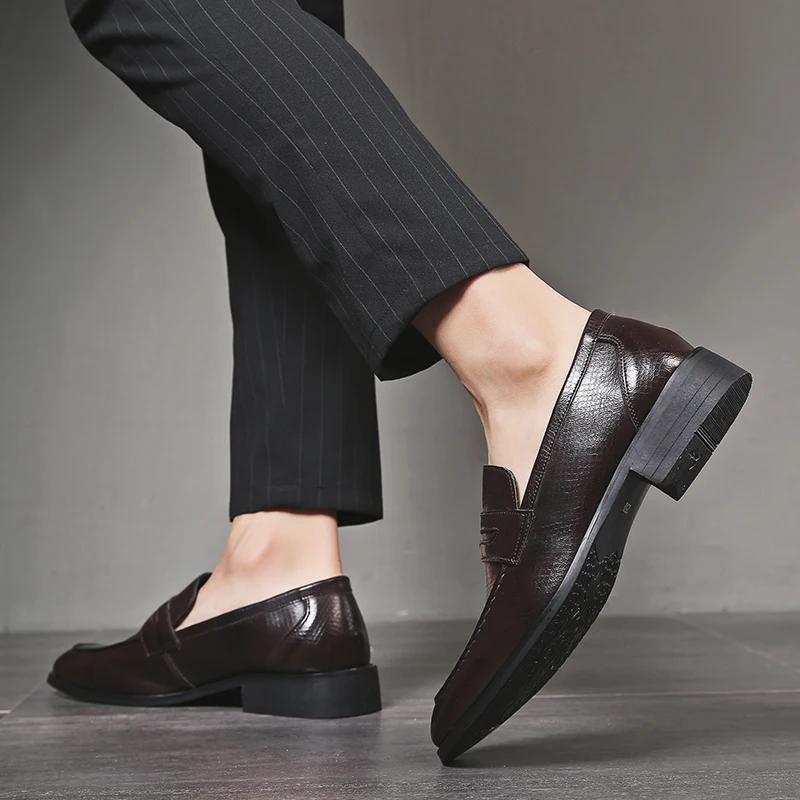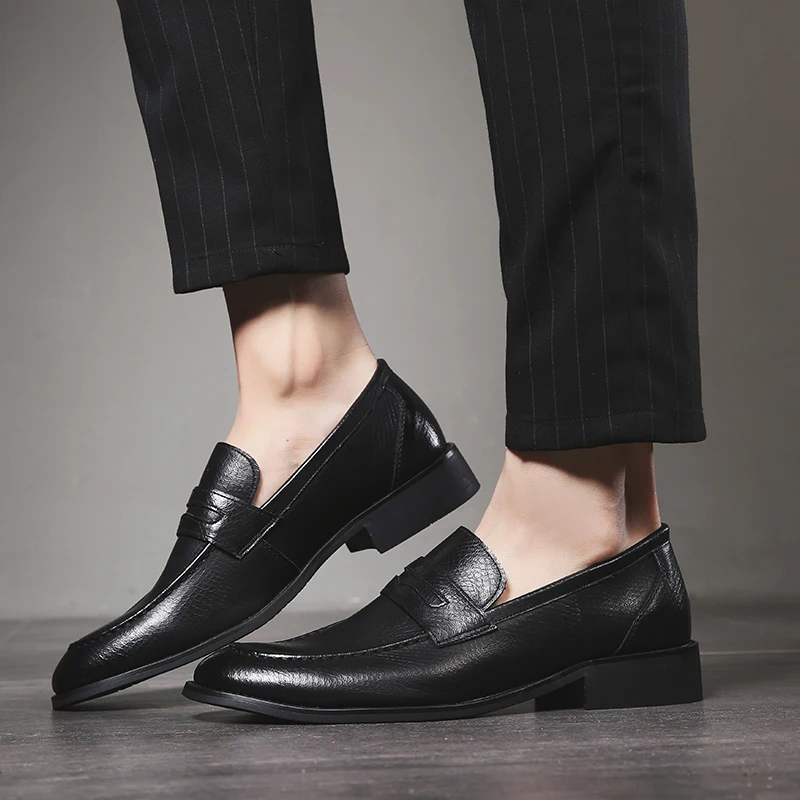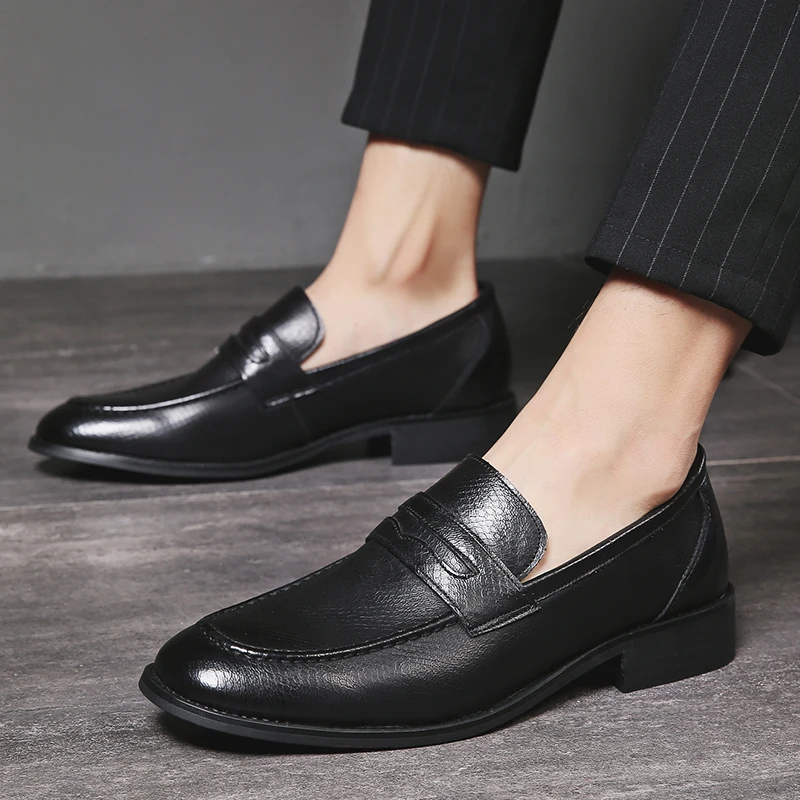PRODUCT DESCRIPTION:
1. Upper Material: Cow Suede
2. Toe Shape: Pointed Toe
3. Outsole Material: Rubber
4. Insole Material: PU
5. Lining Material: PU
6. Fit: Fits true to size, take your normal size